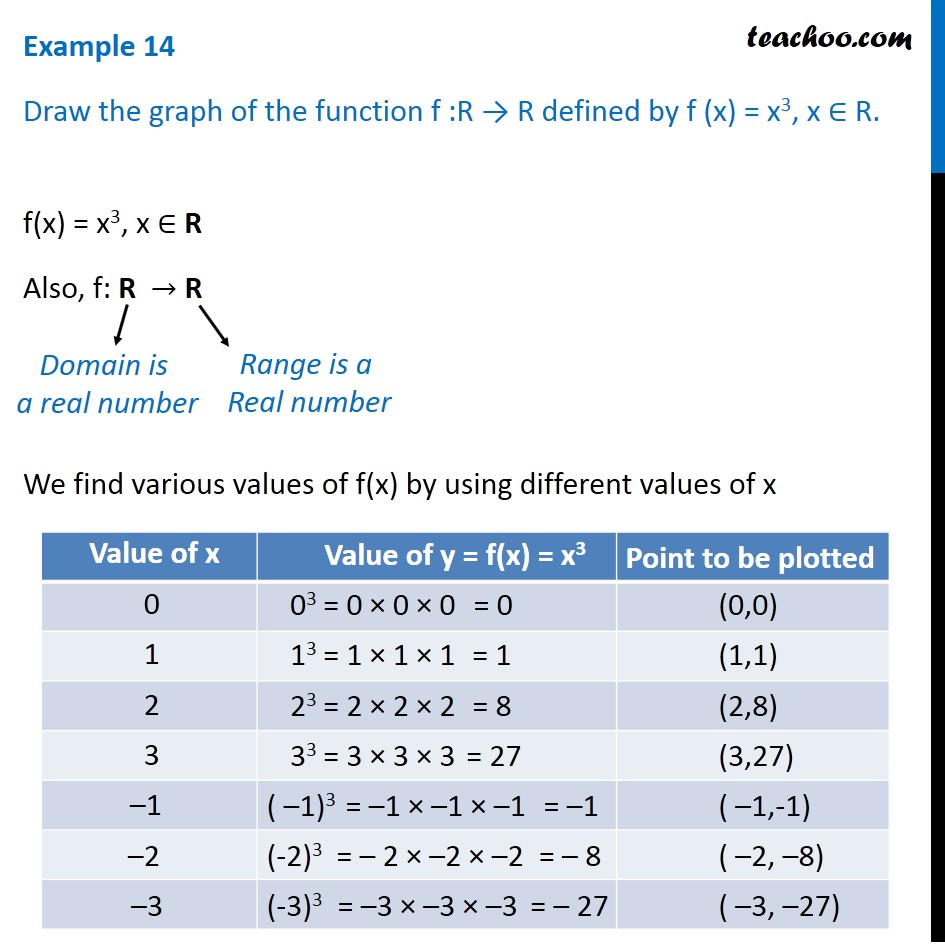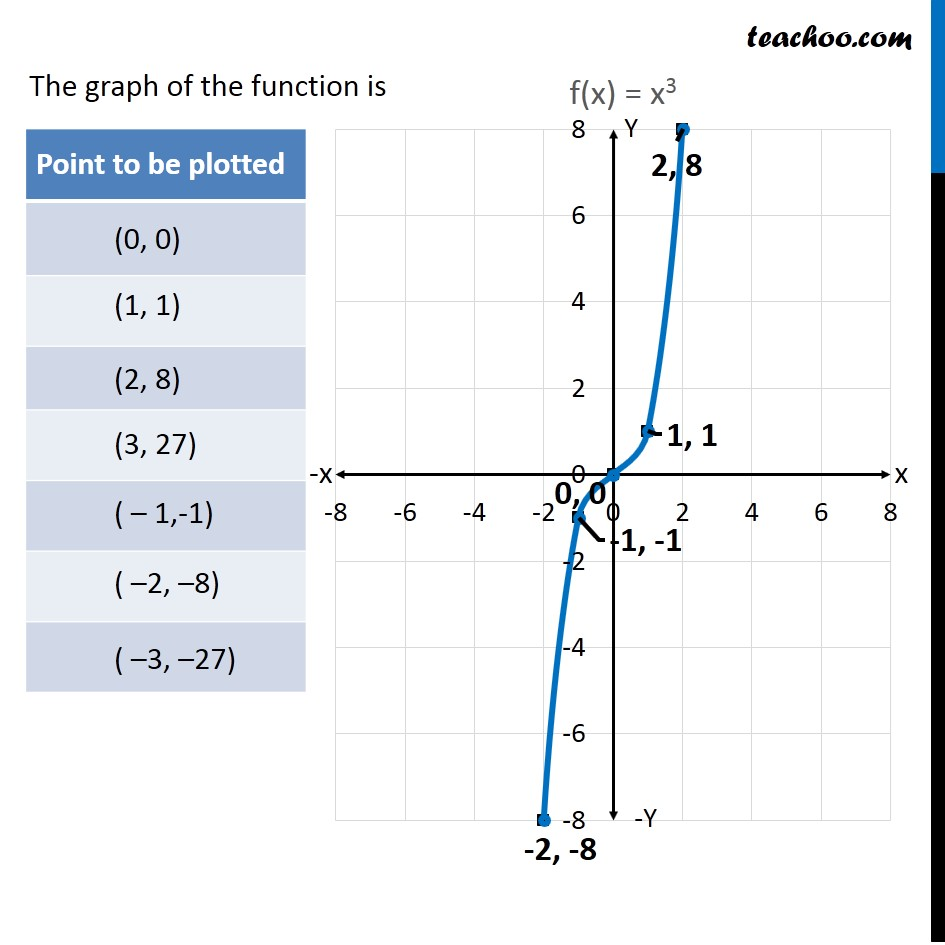Learn All Concepts of Chapter 2 Class 11 Relations and Function - FREE. Check - Relation and Function Class 11 - All Concepts1. Chapter 2 Class 11 Relations and Functions
2. Concept wise
3. Finding Domain and Range - By drawing graphs

Transcript

Example 14 Draw the graph of the function f :R → R defined by f (x) = x3, x ∈ R. f(x) = x3, x ∈ R Also, f: R → R We find various values of f(x) by using different values of x Domain is a real number Range is a Real number The graph of the function is

Finding Domain and Range - By drawing graphs